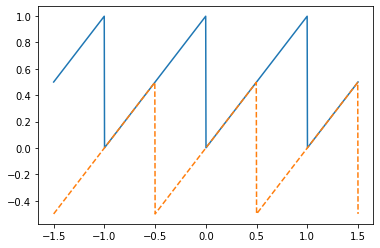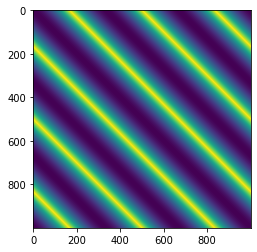# Particle system simulation and fitting¶

This tours shows how to use PyTorch and Keops to compute the evolution of a particles system with a simple interaction energy.

In :
import numpy as np
import matplotlib.pyplot as plt
import torch
import torch.nn as nn
import torch.nn.functional as F
import torch.optim as optim
from time import time
import progressbar


Check if cuda is available (be sure to go to the parameter of the notebook to activate GPU)

In :
device = torch.device("cuda" if torch.cuda.is_available() else "cpu")
print(device)

cuda


Draw random particles.

In :
n = 10000 # number of points
m = 10100 # number of points on the second cloud
d = 2 # dimension
X = torch.rand(n,d)
Y = torch.rand(m,d)
print( X.is_cuda )
X = X.to(device); # put it on gpu
Y = Y.to(device); # put it on gpu
print( X.is_cuda )

False
True


Handling boundary conditions.

In :
boundary = 'no' # no boundary condition
boundary = 'per' # periodic
if boundary=='no': # change this for usual BC
print('No boundary.')
def bc_pos(X): return X
def bc_diff(D): return D
else:
print('Periodic boundary.')
def bc_pos(X): return torch.remainder(X,1.0)
def bc_diff(D): return torch.remainder(D-.5,1.0)-.5

t = torch.tensor(np.linspace(-1.5,1.5,1000))
plt.plot( t, bc_pos( t ) )
plt.plot( t, bc_diff( t ), '--' )

Periodic boundary.

Out:
[<matplotlib.lines.Line2D at 0x7f863a6a8890>]Example of how to compute a pairwise distance matrix $$D_{i,j} = \|x_i-y_j\|^2$$ efficiently using the None keyword.

In :
def distmat_square(X,Y):
return torch.sum( bc_diff(X[:,None,:] - Y[None,:,:])**2, axis=2 )

In :
plt.imshow( distmat_square(t[:,None],t[:,None]) )

Out:
<matplotlib.image.AxesImage at 0x7f863a196290>A more memory efficient way (specially in high dimension $d$) way to compute the distance by expanding the squares.

In :
def distmat_square2(X, Y):
X_sq = (X ** 2).sum(axis=-1)
Y_sq = (Y ** 2).sum(axis=-1)
cross_term = X.matmul(Y.T)
return X_sq[:, None] + Y_sq[None, :] - 2 * cross_term

In :
t0 = time()
distmat_square(X, Y)
print(time() - t0)

t0 = time()
distmat_square2(X, Y)
print(time() - t0)

0.027776718139648438
0.04250526428222656


# Maximum Mean Discrepencies¶

When training ML model with point clouds, it is important to be able to compare to pair of clouds. A simple loss function is to use an MMD norm $$\text{MMD}(X,Y) \triangleq \frac{1}{n^2} \sum_{i,i'} k(x_i,x_{i'}) + \frac{1}{m^2} \sum_{j,j'} k(y_j,y_{j'}) -2 \frac{1}{nm} \sum_{i,j} k(x_i,y_{j}).$$ We use here the energy distance kernel, so that MMD$(X,Y)$ is a dual Sobolev norm between the discrete measure $\frac{1}{n}\sum_i \delta_{x_i}$ and $\frac{1}{m}\sum_i \delta_{y_j}$ $$k(x,y) = -\|x-y\|.$$

In :
def kernel(X,Y):
return -torch.sqrt( distmat_square(X,Y) )

def MMD(X,Y):
n = X.shape
m = Y.shape
a = torch.sum( kernel(X,X) )/n**2 + \
torch.sum( kernel(Y,Y) )/m**2 - \
2*torch.sum( kernel(X,Y) )/(n*m)
return a.item()

In :
print( MMD(X,X) ) # should be 0
print( MMD(X,Y) ) # should be >0

0.0
6.395578384399414e-05


# Gradient flow with Pytorch¶

We compute a speed vector field to advances the particlesusing an interaction kernel of the form $$v(X)_i = \frac{1}{n} \sum_j \psi(\|x_i-x_j\|^2) (x_j-x_i)$$ Note that the evolution $$\dot X = -v(X)$$ is the Wasserstein gradient flow of the energy $$\sum_{i,j} \phi(\|x_i-y_j\|^2 ).$$ when defining $\psi(r)=4\phi'(r)$.

If $\phi$ is decreasing (resp. increasing), the flow is repulsive (resp. attractive).

In :
sigma = .1;
def psi(r):
return torch.exp( -r/(2*sigma**2) )

In :
def Speed(X):
return 2/X.shape * 1/sigma**2 * torch.sum( psi(distmat_square(X,X))[:,:,None] * bc_diff( X[:,None,:] - X[None,:,:] ), axis=1 )


Discretize the evolution $\dot X = -v(X)$.

In :
if boundary=='no':
tau = 1/500 # time step
else:
tau = 1/200
niter = 200
save_per = 10 # periodicity of saving
Zsvg = torch.zeros((n,2,niter//save_per)) # to store all the intermediate time
Z = X
for it in progressbar.progressbar(range(niter)):
if np.mod(it,save_per)==0:
Zsvg[:,:,it//save_per] = Z.clone().detach() # for later display
Z = bc_pos( Z - tau*Speed(Z) )

100% (200 of 200) |######################| Elapsed Time: 0:00:15 Time:  0:00:15


Display the evolution.

In :
import ipywidgets as widgets
@widgets.interact(t=(0,niter//save_per-1))
def display_frame(t=0):
s = t/(niter//save_per-1)
plt.scatter(Zsvg[:,0,t], Zsvg[:,1,t], color=[s,0,1-s])
plt.axis('equal')
plt.axis([0,1,0,1])


# Computing Gradient with Auto-diff¶

Instead of computing "by hand" the gradient of the interaction energy, one can directly rely on Pytorch auto-diff functionality. This simplifies coding and it reduces bugs in code.

In :
X.requires_grad = True
L = -1/X.shape * torch.sum( psi(distmat_square(X,X)), axis=(0,1) )
[g] = torch.autograd.grad(L, [X])
# compare with the "by hand" computation
print( 'Difference "hand" vs. pytorch" : ' + str( torch.norm( g-Speed(X) ).item()  / torch.norm( g ).item() ) )

Difference "hand" vs. pytorch" : 1.7333064950325788e-07


# Computations using Keops¶

In order to speed up (by a factor 10 to 100) the evaluation of the kernel, it is possible to use Keops, which allows one to define "lazy tensor". This both reduces the memory footprint (by evaluating the kernel on the fly) and accelerate the computation by a careful mapping of the tensor entries evaluations on the tiles of the GPU.

In :
!pip install pykeops[colab] > install.log

In :
import pykeops
import pykeops.torch as keops
pykeops.clean_pykeops()          # just in case old build files are still present
pykeops.test_torch_bindings()    # perform the compilation

Cleaning /root/.cache/pykeops-1.5-cpython-37/...
[pyKeOps] Initializing build folder for dtype=float32 and lang=torch in /root/.cache/pykeops-1.5-cpython-37 ... done.
[pyKeOps] Compiling libKeOpstorch180bebcc11 in /root/.cache/pykeops-1.5-cpython-37:
formula: Sum_Reduction(SqNorm2(x - y),1)
aliases: x = Vi(0,3); y = Vj(1,3);
dtype  : float32
...
[pyKeOps] Compiling pybind11 template libKeOps_template_574e4b20be in /root/.cache/pykeops-1.5-cpython-37 ... done.
Done.

pyKeOps with torch bindings is working!



Compute the gradient of a kernel interaction.

In :
X.requires_grad = True
D = keops.Vi(X) - keops.Vj(X)
if boundary=='per':
D1 = (D-.5).mod(1.0)-.5 # for periodic BC
else:
D1 = D
D2 = ( D1 ** 2 ).sum( dim=2 )
K = ( -D2 / (2*sigma**2) ).exp()
L = -1/X.shape * (K.sum(dim=1)**1).sum()
[g] = torch.autograd.grad(L, [X])
# compare with the "by hand" computation
print( 'Difference "hand" vs. "keops+pytorch" : ' + str( torch.norm( g-Speed(X) ).item()  / torch.norm( g ).item() ) )

[pyKeOps] Compiling libKeOpstorchea57037698 in /root/.cache/pykeops-1.5-cpython-37:
formula: Sum_Reduction(Exp((Minus(Sum(Square((Mod(((Var(0,2,0) - Var(1,2,1)) - Var(2,1,2)), Var(3,1,2), IntCst(0)) - Var(4,1,2))))) / Var(5,1,2))),0)
aliases: Var(0,2,0); Var(1,2,1); Var(2,1,2); Var(3,1,2); Var(4,1,2); Var(5,1,2);
dtype  : float32
...
Done.
[pyKeOps] Compiling libKeOpstorch17a5f3fca7 in /root/.cache/pykeops-1.5-cpython-37:
formula: Grad_WithSavedForward(Sum_Reduction(Exp((Minus(Sum(Square((Mod(((Var(0,2,0) - Var(1,2,1)) - Var(2,1,2)), Var(3,1,2), IntCst(0)) - Var(4,1,2))))) / Var(5,1,2))),0), Var(0,2,0), Var(6,1,0), Var(7,1,0))
aliases: Var(0,2,0); Var(1,2,1); Var(2,1,2); Var(3,1,2); Var(4,1,2); Var(5,1,2); Var(6,1,0); Var(7,1,0);
dtype  : float32
...
Done.
[pyKeOps] Compiling libKeOpstorchfdfa2e7b49 in /root/.cache/pykeops-1.5-cpython-37:
formula: Grad_WithSavedForward(Sum_Reduction(Exp((Minus(Sum(Square((Mod(((Var(0,2,0) - Var(1,2,1)) - Var(2,1,2)), Var(3,1,2), IntCst(0)) - Var(4,1,2))))) / Var(5,1,2))),0), Var(1,2,1), Var(6,1,0), Var(7,1,0))
aliases: Var(0,2,0); Var(1,2,1); Var(2,1,2); Var(3,1,2); Var(4,1,2); Var(5,1,2); Var(6,1,0); Var(7,1,0);
dtype  : float32
...
Done.
Difference "hand" vs. "keops+pytorch" : 2.946057011364191e-07

In :
Zsvg = torch.zeros((n,2,niter//save_per)) # to store all the intermediate time
Z = X
Z.requires_grad = True
for it in progressbar.progressbar(range(niter)):
if np.mod(it,save_per)==0:
Zsvg[:,:,it//save_per] = Z.clone().detach() # for later display
D = keops.Vi(Z) - keops.Vj(Z)
if boundary=='per':
D1 = (D-.5).mod(1.0)-.5 # for periodic BC
else:
D1 = D
D2 = ( D1 ** 2 ).sum( dim=2 )
K = ( -D2 / (2*sigma**2) ).exp()
L = 1/X.shape * (K.sum(dim=1)**1).sum() # There is a bug, I needed to add **1 here !!
[g] = torch.autograd.grad(L, [Z])
Z = bc_pos( Z + tau*g )

100% (200 of 200) |######################| Elapsed Time: 0:00:01 Time:  0:00:01

In :
import ipywidgets as widgets
@widgets.interact(t=(0,niter//save_per-1))
def display_frame(t=0):
s = t/(niter//save_per-1)
plt.scatter(Zsvg[:,0,t], Zsvg[:,1,t], color=[s,0,1-s])
plt.axis('equal')
plt.axis([0,1,0,1])

In :